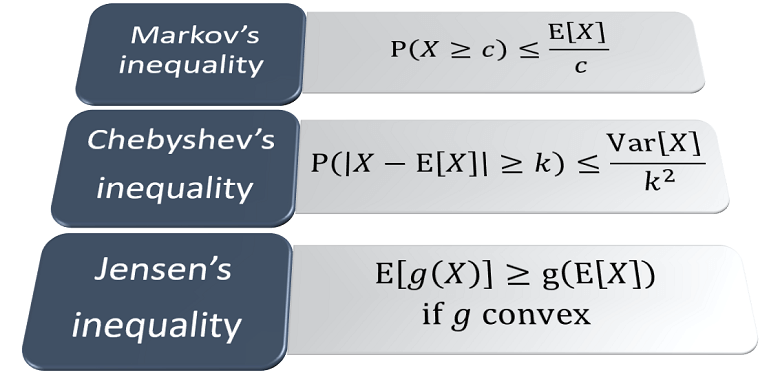StatLect

# Fundamentals of probability theory

This is an introduction to the fundamental concepts of probability theory. Each lecture contains detailed proofs and derivations of all the main results, as well as solved exercises.

## Probability and events

Events having zero probability, almost sure events, almost sure properties

Sample space, sample points, events, probability and its fundamental properties

Prior probability, posterior probability, updating

How to revise probabilities when new information arrives

Definition and explanation of independence and mutual independence

## Random variables and random vectors

Joint distributions, marginal distributions

Discrete and continuous random variables, probability mass and density functions

A rigorous definition of expected value, based on the theory of Lebesgue integration

The mean of a random variable, how to compute it, its properties

Dispersion around the mean, definition, interpretation, fundamental properties

Linearity of the expected value, expectation of positive random variables, other properties

Another measure of association between random variables

Association between random variables, definition, interpretation, properties

Equal to one when an event happens and zero otherwise

A multivariate generalization of the concept of variance

Cut-off point of a distribution that leaves to its left a given proportion of the distribution

## Conditional distributions and independence

How to update the distribution of a random variable after receiving some information

A more rigorous presentation of conditional probability based on the theory of sigma-algebras

Definition and characterization of independence between random variables

How to compute the expected value of a random variable after observing the value of another

## Inequalities

A fundamental inequality derived from Markov's inequality

Provides an upper bound to the probability that a random variable will exceed a threshold

Concerns the expected value of convex and concave transformations of a random variable## More about probability mass and density functions

Properties of probability density functions and how to construct them

Properties of probability mass functions and how to construct them

Factorization into marginal and conditional probability density function

Factorization into marginal and conditional probability mass function

## Transformations of random variables

How to derive the joint distribution of a function of a random vector

How to derive the distribution of Y=g(X) from the distribution of X

How to derive the distribution of a sum from the distributions of the summands

## Moments

Definition of cross-moment and central cross-moment of a random vector

Definition of moment and central moment of a random variable

## Moment generating and characteristic functions

Generalizes the concept of moment generating function to random vectors

Definition, computation of moments, characterization of distributions

Generalizes the concept of characteristic function to random vectors

Definition, computation of moments, characterization of distributions

The logarithm of the moment generating function has some interesting properties

## Information-theoretic concepts

A measure of the dissimilarity between two probability distributions

## Revise what you studied

200 probability questions to check your knowledge of probability theory

The books

Most of the learning materials found on this website are now available in a traditional textbook format.

Glossary entries
Share